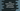# Java Math.random() method example to create random numbers## Java Math.random() method explanation with example:

java.lang.Math class provides different utility functions and constants. random() is a method defined in the Math class and this method is used to generate random numbers.

In this post, we will learn about the random() method with examples.

### Definition of random():

The random() method is defined in the Math class and it is defined as like below:

``public static double random​()``

As you can see here, it is a public static method and it returns a double value. So, we can call this method without creating any object of the Math class.

This method returns one random positive value in between 0.0 inclusive to 1.0 exclusive.

It creates a new pseudorandom number generator when we first call it by calling new java.util.Random(). This generator is used for all calls to the random method after that.

Let’s take a look at an example:

### Example of Math.random():

``````public class Main {
public static void main(String[] args) {
System.out.println(Math.random());
System.out.println(Math.random());
}
}``````

In this program, I am using Math.random() two times and printing its values. It will print something like below:

``````0.06777530972426915
0.007258937778229946``````

Each time you run the program, it will print a different result.

### Random values at a range:

We can also use the random method to print random values at any range. For example, if we want random numbers in between lowerRange and upperRange, then we have to use:

``(int)(Math.random() * (upperRange - lowerRange + 1)) + lowerRange``

For example,

``````public class Main {
public static int getRandom(int lowerRange, int upperRange){
return (int)(Math.random() * (upperRange - lowerRange + 1)) + lowerRange;
}
public static void main(String[] args) {
System.out.println(getRandom(1, 100));
}
}``````

In this program, getRandom method will return one random number between lowerRange and upperRange and both are inclusive.

This program will print a random value between 1 to 100 each time you execute it.